In Online Education NCERT Solutions for Class 10 Maths Chapter 10 Circles Ex 10.1 are part of NCERT Solutions for Class 10 Maths. Here we have given NCERT Solutions for Class 10 Maths Chapter 10 Circles Ex 10.2.

 Board CBSE Textbook NCERT Class Class 10 Subject Maths Chapter Chapter 10 Chapter Name Circles Exercise Ex 10.2 Number of Questions Solved 13 Category NCERT Solutions

## Online Education NCERT Solutions for Class 10 Maths Chapter 10 Circles Ex 10.2

Question 1.
From a point Q, the length of the tangent to a circle is 24 cm and the distance of Q from the centre is 25 cm. The radius of the circle is
(a) 7 cm Sol.
(b) 12 cm
(c) 15 cm
(d) 24.5 cm
Solution: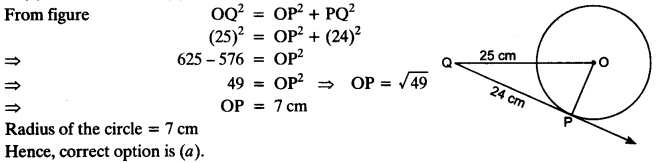Question 2.
In figure, if TP and TQ are the two tangents to a circle with centre O so that ∠POQ = 110°, then ∠PTQ is equal to
(a) 60°
(b) 70°
(c) 80°
(d) 90°
Solution: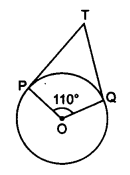∠OPT = 90°
∠OQT = 90°
∠POQ = 110°
∴ ∠PTQ + ∠POQ = 180° ⇒ ∠PTQ + 110° = 180°
⇒∠PTQ = 180°- 110° = 70°
Hence, correct option is (b).

Question 3.
If tangents PA and PB from a point P to a circle with centre O are inclined to each other at angle of 80°, then ∠POA is equal to
(a) 50°
(b) 60°
(c) 70°
(d) 80°
Solution:
In AOAP and AOBP
PA = PB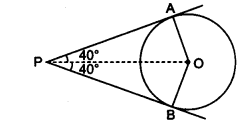[Lengths of tangents from an external point are equal]
OP = OP [Common]
∴ ∆OAP ≅ ∆OBP [SSS congruence rule]
∠AOB + ∠APB = 180° ⇒ ∠AOB + 80° = 180°
⇒∠AOB = 180° – 80° = 100°
From eqn. (i), we get
⇒∠POA = $$\frac { 1 }{ 2 }$$ x 100° = 50°
Hence, correct option is (a)

Question 4.
Prove that the tangents drawn at the ends of a diameter of a circle are parallel.
Solution:
AB is a diameter of the circle, p and q are two tangents.
OA ⊥ p and OB ⊥ q
∠1 = ∠2 = 90°
⇒ p || q ∠1 and ∠2 are alternate angles]Question 5.
Prove that the perpendicular at the point of contact to the tangent to a circle passes through the centre.
Solution:
XY tangent to the circle C(0, r) at B and AB ⊥ XY. Join OB.
∠ABY = 90° [Given]
∠OBY = 90°
[Radius through point of contact is perpendicular to the tangent]
∴ ∠ABY + ∠OBY = 180° ⇒ ABOiscollinear
∴ AB passes through centre of the circle.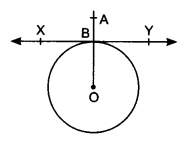Question 6.
The length of a tangent from a point A at distance 5 cm from the centre of the circle is 4 cm. Find the radius of the circle.
Solution:
OA = 5 cm, AP = 4 cm OP = Radius of the circle
∠OPA = 90° [Radius and tangent are perpendicular]Question 7.
Two concentric circles are of radii 5 cm and 3 cm. Find the length of the chord of the larger circle which touches the smaller circle.
Solution:
Radius of larger circle = 5 cm Radius of smaller circle = 3 cm
OP ⊥ AB
[Radius of circle is perpendicular to the tangent]
AB is a chord of the larger circleQuestion 8.
A quadrilateral ABCD is drawn to circumscribe a circle (see figure). Prove that AB + CD = AD + BC.
Solution:
AP = AS … (i)
[Lengths of tangents from an external point are equal]
BP = BQ … (ii)
CR = CQ … (iii)
DR = DS … (iv)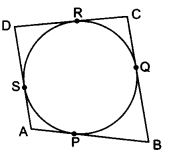Adding equations (i), (ii), (iii) and (iv), we get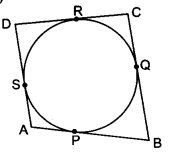AP + BP + CR + DR = AS + BQ + CQ + DS
⇒ (AP + BP) + (CR + DR) = (AS + DS) + (BQ + CQ)
⇒ AB + CD = AD + BC
Hence proved.

Question 9.
In figure, XY and X’Y’ are two parallel tangents to a circle , x with centre O and another tangent AB with point of contact C intersecting XY at A and X’Y’ at B. Prove that ∠AOB = 90°.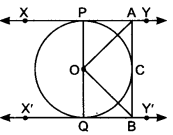Solution:
Given: Two parallel tangents to a circle with centre O. Tangent AB with point of contact C intersects XY at A and X’Y’ at B To Prove: ∠AOB = 90° with point of contact C intersects XY at A and X’Y’ at BTo Prove: ∠AOB = 90°
Construction: Join OA, OB and OC
Proof: In ∆AOP and ∆AOCQuestion 10.
Prove that the angle between the two tangents drawn from an external point to a circle is supplementary to the angle subtended by the line segment joining the points of contact at the centre.
Solution:
PA and PB are two tangents, A and B are the points of contact of the tangents.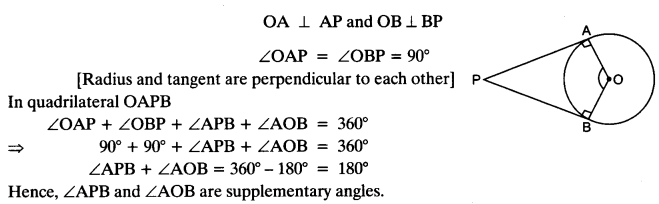Question 11.
Prove that the parallelogram circumscribing a circle is a rhombus.
Solution:
Parallelogram ABCD circumscribing a circle with centre O.
OP ⊥ AB and OS ⊥ ADQuestion 12.
A triangle ABC is drawn to circumscribe a circle of radius 4 cm such that the segments BD and DC into which BC is divided by the point of contact D are of lengths 8 cm and 6 cm respectively (see figure). Find the sides AB and AC.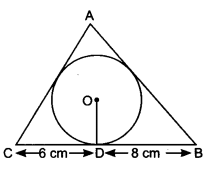Solution:
BD = 8 cm and DC = 6 cm
BE = BD = 8 cm
CD = CF = 6 cm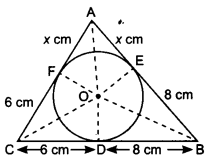Let AE = AF = x cm
In ∆ABC, a = 6 + 8 = 14 cm
b = (x + 6) cm
c = (x + 8) cmQuestion 13.
Prove that opposite sides of a quadrilateral circumscribing a circle subtend supplementary angles at the centre of the circle.
Solution:
AB touches at P.
BC, CD and DA touch the circle at Q, R and S.
Construction: Join OA, OB, OC, OD and OP, OQ, OR, OS.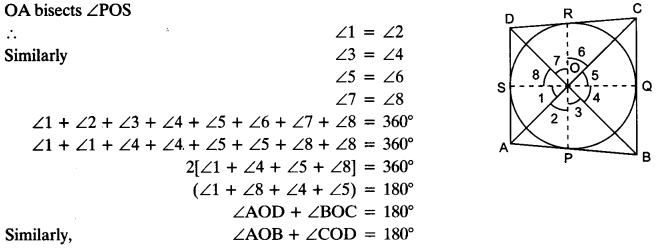Hence, opposite sides of quadrilateral circumscribing a circle subtend supplementary angles at the centre of a circle.

We hope the NCERT Solutions for Class 10 Maths Chapter 10 Circles Ex 10.2, help you. If you have any query regarding NCERT Solutions for Class 10 Maths Chapter 10 Circles E 10.2, drop a comment below and we will get back to you at the earliest.